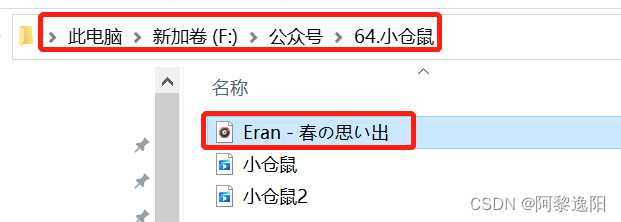## 二、代码详解

Python绘制小仓鼠的原理是：应用turtle库绘制身体的不同部位。

### 1 导入库

``````# -*- coding: UTF-8 -*-
'''

'''
import os
import pygame
import turtle as t
``````

os库可以设置文件读取的位置。

pygame库是为了绘制过程更有趣，在绘图过程中添加了背景音乐。

turtle库是绘图库，相当于给你一支画笔，你可以在画布上用数学逻辑控制的代码完成绘图。

### 2 播放音乐

``````#播放音乐
print('播放音乐')
pygame.mixer.init()
pygame.mixer.music.set_volume(0.5)
pygame.mixer.music.play(1, 10)
``````### 3 定义画小仓鼠头的函数

``````t.title('阿黎逸阳的代码公众号')
t.speed(10)
#t.screensize(1000, 800)
t.setup(startx=0, starty = 0, width=800, height = 600)
print('画右耳朵')
#画右耳朵
t.penup()
t.goto(100, 150)
t.pendown()
t.pensize(0.5)
t.color('cornsilk')
#t.color('cornsilk', 'cornsilk')
t.begin_fill()
t.circle(40, 20)
t.circle(7, 180)
t.right(22)
t.circle(50, 12)
print('画头')
#画头
t.circle(200, 14)
print('画左耳朵')
#画左耳朵
t.circle(40, 20)
t.circle(7, 180)
t.right(22)
t.circle(50, 12)
print('画左脸')
#画左脸
t.circle(200, 10)
t.circle(20, 100)
print('画脸上的波浪线')
#画脸上的波浪线
t.circle(-30, 100)
t.circle(-10, 130)
t.circle(-10, 130)
t.circle(-38, 100)
t.circle(20, 130)
t.circle(80, 22)
t.end_fill()
``````

t.pensize(width)：设置画笔的尺寸。

t.color(color)：设置画笔的颜色。

t.penup()：抬起画笔，一般用于另起一个地方绘图使用。

t.goto(x,y)：画笔去到某个位置，参数为(x,y)，对应去到的横坐标和纵坐标。

t.pendown()：放下画笔，一般和penup组合使用。

t.left(degree)：画笔向左转多少度，括号里表示度数。

t.right(degree)：画笔向右转多少度，括号里表示度数。

### 4 定义画左眼和右眼的函数

``````print('画左眼睛')
#画左眼睛
t.penup()
t.goto(30, 115)
t.pendown()
t.color('black')
t.begin_fill()
t.circle(-20, 40)
t.circle(6, 205)
t.end_fill()
print('画右眼睛')
#画右眼睛
t.penup()
t.goto(90, 116)
t.pendown()
t.color('black')
t.begin_fill()
t.circle(20, 40)
t.circle(-6, 205)
t.end_fill()
``````

### 5 定义画嘴的函数

``````#画嘴巴
t.color('black', 'yellow')
t.begin_fill()
t.circle(10, 60)
t.circle(-30, 30)
t.circle(-9, 180)
t.left(20)
t.circle(-50, 12)
t.circle(10, 60)
t.end_fill()
``````

Python人脸识别—我的眼里只有你
Python画好看的星空图（唯美的背景）
【Python】情人节表白烟花（带声音和文字）

Python浪漫表白源码合集（爱心、玫瑰花、照片墙、星空下的告白）